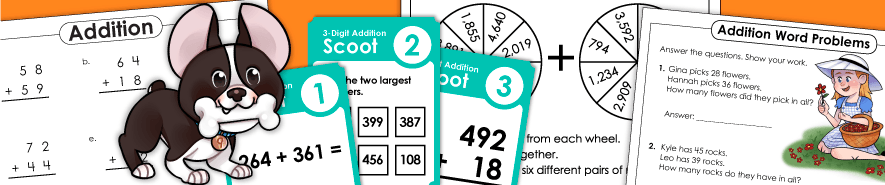The links below feature a variety of activities for teaching multi-digit addition. Print worksheets, task cards, and games. Skills include 3-digit, 4-digit and 5-digit addends, as well as decimal, money, and fraction addition.The double-digit addition worksheets on this page require students to carry ones, or regroup. Includes graph paper math, a Scoot! game, and word problem worksheets.

These printable worksheets and games have addition problems with 3-digit addends. Includes math riddles, a magic digit game, math crossword puzzles, and column addition worksheets.

Use these printable resources to learn, review, and practice addition with 5-digit addends.

When you print these activities, you'll notice that the problems have 3, 4, or 5 addends. Includes 1, 2, 3, and 4-digit numbers.

Adding 10 and Multiples of 10

## Adding Decimals, Money, and Fractions

On these worksheets, students will practice adding fractions and mixed numbers. Includes fractions with same denominator and different denominators.
Approx. levels: 3rd, 4th, 5th, and 6th grades

Find sums and differences for pairs of decimals on these worksheets. These practice pages have decimals in tenths, hundredths, and thousandths.

These printables have horizontal and vertical money addition problems, as well as word problems.

(Positive and Negative Numbers)

Practice finding sums and differences of positive and negative integers.

Learn about the commutative and associative properties of addition with these printables.
Approx. levels: 3rd, 4th, 5th

These basic addition problems have addends up to 5 and sums up to 10. Includes puzzles, practice worksheets, card games, illustrated concept sheets, and more. (examples: 4+1=5, 2+3=5)
Approx. level: Kindergarten and 1st Grades

This page has lots of activities to use when teaching basic addition facts. Includes a memory match game, dice games, bingo, drill worksheets, flashcards, number line practice, and much more. All facts have addends between 0 and 10. (examples: 9+8=17, 8+6=14)
Approx. levels: 1st and 2nd grades

The worksheets you'll find here have a mix of basic addition and subtraction on them.

Basic Addition: Making 10 (Sums of 10)

These worksheets and activities will help students discover pairs of addends with sums of 10.

Learn basic addition doubles facts. These are addition problems in which you add a number to itself.
(examples: 3+3=6, 5+5=10, 7+7=10)

The worksheets on this page can be used to teach students to solve doubles +1 facts. These are addition problems where students double a number, then add one.
(examples: 2+3=5, 5+6=11, 8+9=17)

This section has several worksheets for teaching fact families. (Also known as number bonds or number families.) Approx. levels: 1st and 2nd grades

Sum Game

This page has many different versions of STW's Addition Sum Game. In this game, students take turns circling pairs of numbers that add up to a given amount. For example, they might search for pairs of numbers that add up to 17.

Can your students solve these tricky logic puzzles? There are three levels: basic, intermediate, and advanced.

Math Riddle Worksheets

There are many addition worksheets on our Math Riddle page too. On these sheets, students can decode the answer to a funny riddle by completing arithmetic problems.

Math Mystery Pictures

This section of the site links to many basic, single-digit addition worksheets. Students solve addition problems, then color the mystery picture according to the color-coded key at the bottom of the page.

Multiple Step Word Problems

Students use addition, subtraction, multiplication, and/or division to solve these multi-step math word problems.

Using Number Lines

Learn to add and subtract with number lines.